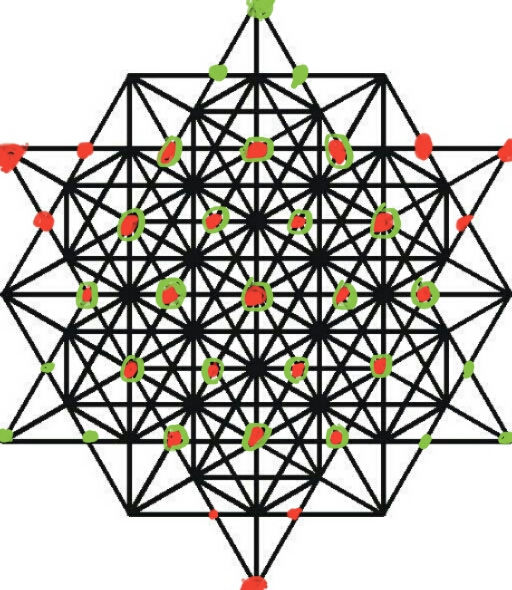top of page
Search

# Isotropic vector matrix(64 tetrahedron grid), 7 tetractys,infinite tetrahedron grid and 168

444=(64 tetrahedron grid(8 tetragrammatons/tetractys/star tetrahedron/tree of life)=(7 tetractys)A 3d 64 tetrahedron grid is made out of 2 3d(7 tetractys) A.K.A (7 tetrahedral tetractys) that are combined to create a star tetrahedron

The star tetrahedron will be made out of 2 (7 tetrahedral tetractys) which are each made out of 84 tetrahedrons so it will be:

84+84=168

There are 168 whorls in a superstring

A superstring is a infinite tetrahedron grid made out of energy in the form of a wave(frequency/vibration)

Triangle numbers(tetractys) added up to create tetrahedral numbers(tetrahedral tetractys):

1+3+6+10+15+21+28=84

The 3d seed of life is made out of 13 spheres and when you join all there centers together you get the vector equilibrium